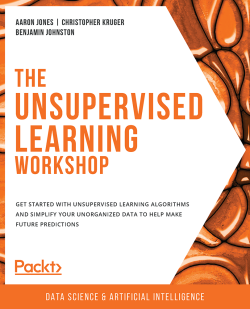# The Unsupervised Learning Workshop

By Aaron Jones , Christopher Kruger , Benjamin Johnston
• \$28.99 eBook
• \$41.99 Print + eBook
What do you get with a Packt Subscription?

• Constantly updated with 100+ new titles each month
• Breadth and depth in over 1,000+ technologies

Do you find it difficult to understand how popular companies like WhatsApp and Amazon find valuable insights from large amounts of unorganized data? The Unsupervised Learning Workshop will give you the confidence to deal with cluttered and unlabeled datasets, using unsupervised algorithms in an easy and interactive manner.

The book starts by introducing the most popular clustering algorithms of unsupervised learning. You'll find out how hierarchical clustering differs from k-means, along with understanding how to apply DBSCAN to highly complex and noisy data. Moving ahead, you'll use autoencoders for efficient data encoding.

As you progress, you’ll use t-SNE models to extract high-dimensional information into a lower dimension for better visualization, in addition to working with topic modeling for implementing natural language processing (NLP). In later chapters, you’ll find key relationships between customers and businesses using Market Basket Analysis, before going on to use Hotspot Analysis for estimating the population density of an area.

By the end of this book, you’ll be equipped with the skills you need to apply unsupervised algorithms on cluttered datasets to find useful patterns and insights.

Publication date:
July 2020
Publisher
Packt
Pages
550
ISBN
9781800200708

# 1. Introduction to Clustering

Overview

Finding insights and value in data is the ambitious promise that has been seen in the rise of machine learning. Within machine learning, there are predictive approaches to understanding dense information in deeper ways, as well as approaches to predicting outcomes based on changing inputs. In this chapter, we will learn what supervised learning and unsupervised learning are, and how they are applied to different use cases. Once you have a deeper understanding of where unsupervised learning is useful, we will walk through some foundational techniques that provide value quickly.

By the end of this chapter, you will be able to implement k-means clustering algorithms using built-in Python packages and calculate the silhouette score.

# Introduction

Have you ever been asked to take a look at some data and came up empty handed? Maybe you weren't familiar with the dataset, or maybe you didn't even know where to start. This may have been extremely frustrating, and even embarrassing, depending on who asked you to take care of the task.

You are not alone, and, interestingly enough, there are many times the data itself is simply too confusing to be made sense of. As you try and figure out what all those numbers in your spreadsheet mean, you're most likely mimicking what many unsupervised algorithms do when they try to find meaning in data. The reality is that many unprocessed real-world datasets may not have any useful insights. One example to consider is the fact that these days, individuals generate massive amounts of granular data on a daily basis – whether it's their actions on a website, their purchase history, or what apps they use on their phone. If you were to look at this information on the surface, it would be a big, unorganized mess with no hope of clarity. Don't fret, however; this book will prepare you for such tall tasks so that you'll never be frustrated again when dealing with data exploration tasks, no matter how large.

For this book, we have developed some best-in-class content to help you understand how unsupervised algorithms work and where to use them. We'll cover some of the foundations of finding clusters in your data, how to reduce the size of your data so it's easier to understand, and how each of these sides of unsupervised learning can be applied in the real world. We hope you will come away from this book with a strong real-world understanding of unsupervised learning, the problems that it can solve, and those it cannot.

# Unsupervised Learning versus Supervised Learning

Unsupervised learning is the field of practice that helps find patterns in cluttered data and is one of the most exciting areas of development in machine learning today. If you have explored machine learning bookwork before, you are probably familiar with the common breakout of problems in either supervised or unsupervised learning. Supervised learning encompasses the problem set of having a labeled dataset that can be used to either classify data (for example, predicting smokers and non-smokers, if you're looking at a lung health dataset) or finding a pattern in clearly defined data (for example, predicting the sale price of a home based on how many bedrooms it has). This model most closely mirrors an intuitive human approach to learning.

For example, if you wanted to learn how to not burn your food with a basic understanding of cooking, you could build a dataset by putting your food on the burner and seeing how long it takes (input) for your food to burn (output). Eventually, as you continue to burn your food, you will build a mental model of when burning will occur and how to avoid it in the future. Development in supervised learning was once fast paced and valuable, but it has simmered down in recent years. Many of the obstacles around getting to know your data have already been tackled and are listed in the following image: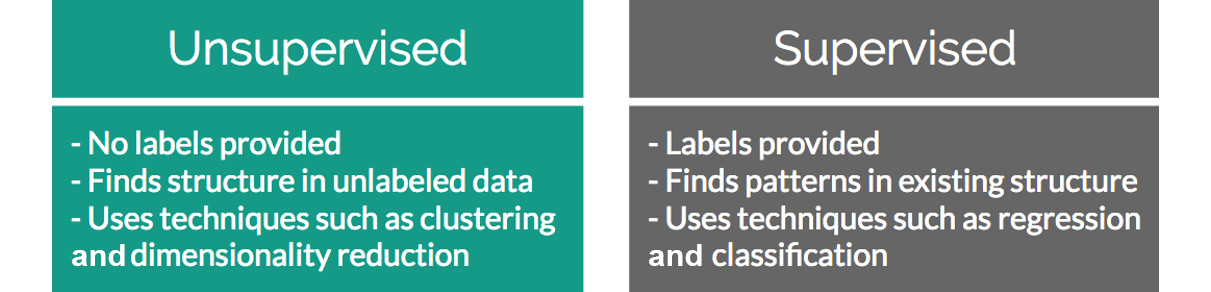Figure 1.1: Differences between unsupervised and supervised learning

Conversely, unsupervised learning encompasses the problem set of having a tremendous amount of data that is unlabeled. Labeled data, in this case, would be data that has a supplied "target" outcome that you are trying to find the correlation to with supplied data. For instance, in the preceding example, you know that your "target outcome" is whether your food was burned; this is an example of labeled data. Unlabeled data is when you do not know what the "target" outcome is, and you have only supplied input data.

Building upon the previous example, imagine you were just dropped on planet Earth with zero knowledge of how cooking works. You are given 100 days, a stove, and a fridge full of food without any instructions on what to do. Your initial exploration of a kitchen could go in infinite directions. On day 10, you may finally learn how to open the fridge; on day 30, you may learn that food can go on the stove; and after many more days, you may unwittingly make an edible meal. As you can see, trying to find meaning in a kitchen devoid of adequate informational structure leads to very noisy data that is completely irrelevant to actually preparing a meal.

Unsupervised learning can be an answer to this problem. Looking back at your 100 days of data, you can use clustering to find patterns of similar attributes across days and deduce which foods are similar and may lead to a "good" meal. However, unsupervised learning isn't a magical answer. Simply finding clusters can be just as likely to help you find pockets of similar, yet ultimately useless, data. Expanding on the cooking example, we can illustrate this shortcoming with the concept of the "third variable". Just because you have a cluster of really great recipes doesn't mean they are infallible. During your research, you may have found a unifying factor that all good meals were cooked on a stove. This does not mean that every meal cooked on a stove will be good, and you cannot easily jump to that conclusion for all future scenarios.

This challenge is what makes unsupervised learning so exciting. How can we find smarter techniques to speed up the process of finding clusters of information that are beneficial to our end goals? The following sections would help us answer this question.

# Clustering

Clustering is the overarching process that involves finding groups of similar data that exist in your dataset, which can be extremely valuable if you are trying to find its underlying meaning. If you were a store owner and you wanted to understand which customers are more valuable without a set idea of what valuable is, clustering would be a great place to start to find patterns in your data. You may have a few high-level ideas of what denotes a valuable customer, but you aren't entirely sure in the face of a large mountain of available data. Through clustering, you can find commonalities among similar groups in your data. For example, if you look more deeply at a cluster of similar people, you may learn that everyone in that group visits your website for longer periods of time than others. This can show you what the value is and also provide a clean sample size for future supervised learning experiments.

## Identifying Clusters

The following image shows two scatterplots:Figure 1.2: Two distinct scatterplots

The following image separates the two scatterplots into two distinct clusters: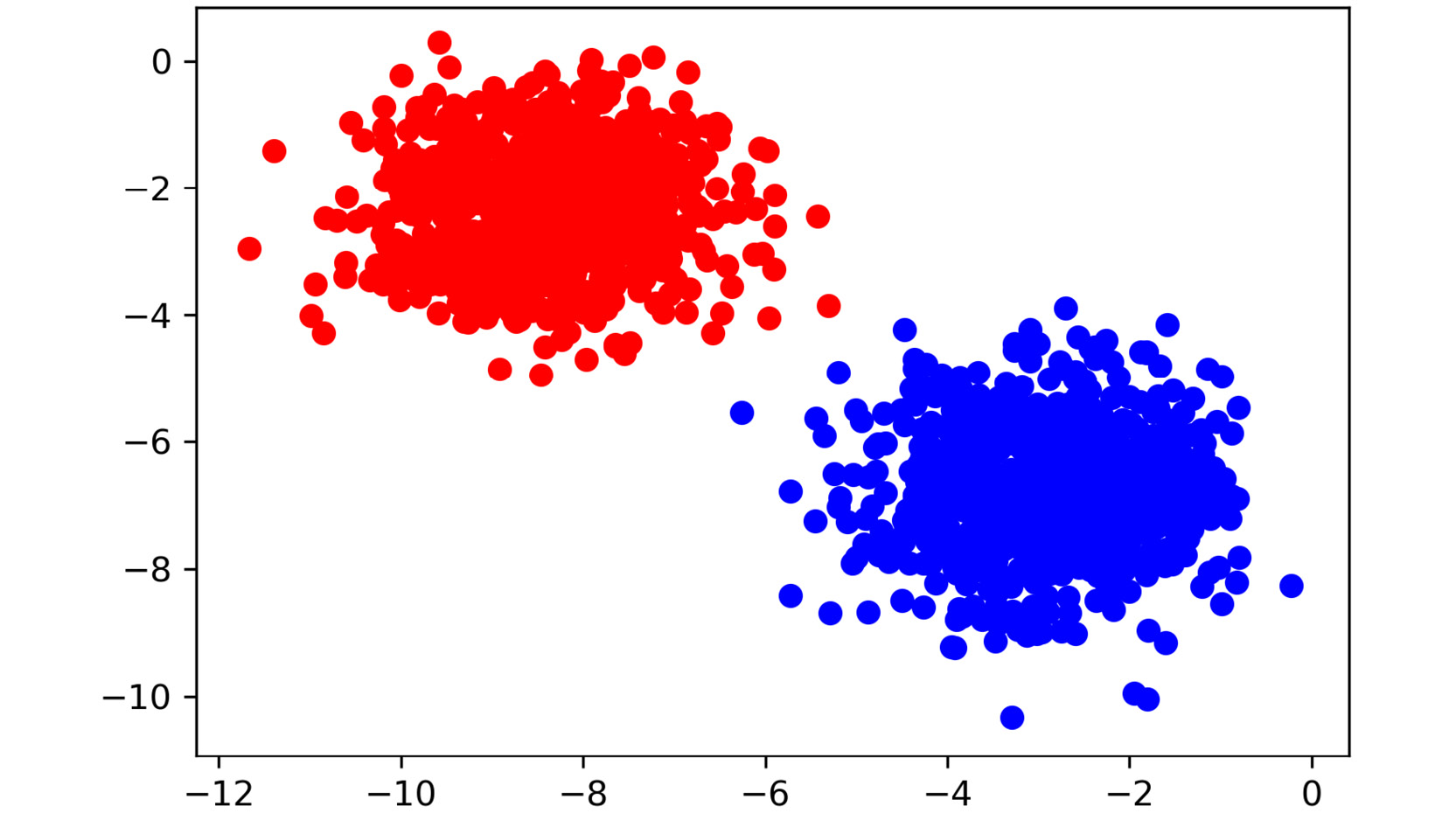Figure 1.3: Scatterplots clearly showing clusters that exist in a provided dataset

Figure 1.2 and Figure 1.3 display randomly generated number pairs (x and y coordinates) pulled from two distinct Gaussian distributions centered at different locations. Simply by glancing at the first image, it should be obvious where the clusters exist in your data; in real life, it will never be this easy. Now that you know that the data can be clearly separated into two clusters, you can start to understand what differences exist between the two groups.

Rewinding a bit from where unsupervised learning fits into the larger machine learning environment, let's begin by understanding the building blocks of clustering. The most basic definition finds clusters simply as groupings of similar data as subsets of a larger dataset. As an example, imagine that you had a room with 10 people in it and each person had a job either in finance or as a scientist. If you told all the financial workers to stand together and all the scientists to do the same, you would have effectively formed two clusters based on job types. Finding clusters can be immensely valuable in identifying items that are more similar and, on the other end of the scale, quite different from one another.

## Two-Dimensional Data

To understand this, imagine that you were given a simple 1,000-row dataset by your employer that had two columns of numerical data, as follows: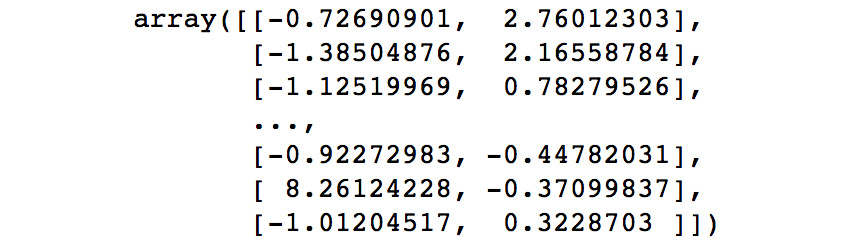Figure 1.4: Two-dimensional raw data in an array

At first glance, this dataset provides no real structure or understanding.

A dimension in a dataset is another way of simply counting the number of features available. In most organized data tables, you can view the number of features as the number of columns. So, using the 1,000-row dataset example of size (1,000 x 2), you will have 1,000 observations across two dimensions. Please note that dimensions of dataset should not be confused with the dimensions of an array.

You begin by plotting the first column against the second column to get a better idea of what the data structure looks like. There will be plenty of times where the cause of differences between groups will prove to be underwhelming; however, the cases that have differences that you can take action on are extremely rewarding.

## Exercise 1.01: Identifying Clusters in Data

You are given two-dimensional plots of data that you suspect have clusters of similar data. Please look at the two-dimensional graphs provided in the exercise and identify the groups of data points to drive the point home that machine learning is important. Without using any algorithmic approaches, identify where these clusters exist in the data.

This exercise will help you start building your intuition of how we can identify clusters using our own eyes and thought processes. As you complete this exercise, think of the rationale of why a group of data points should be considered a cluster versus a group that should not be considered a cluster. Follow these steps to complete this exercise:

1. Identify the clusters in the following scatterplot: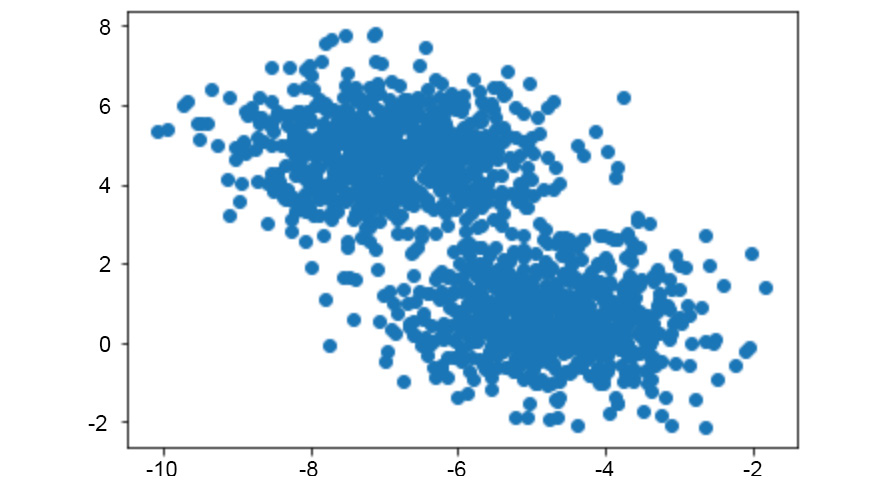Figure 1.5: Two-dimensional scatterplot

The clusters are as follows: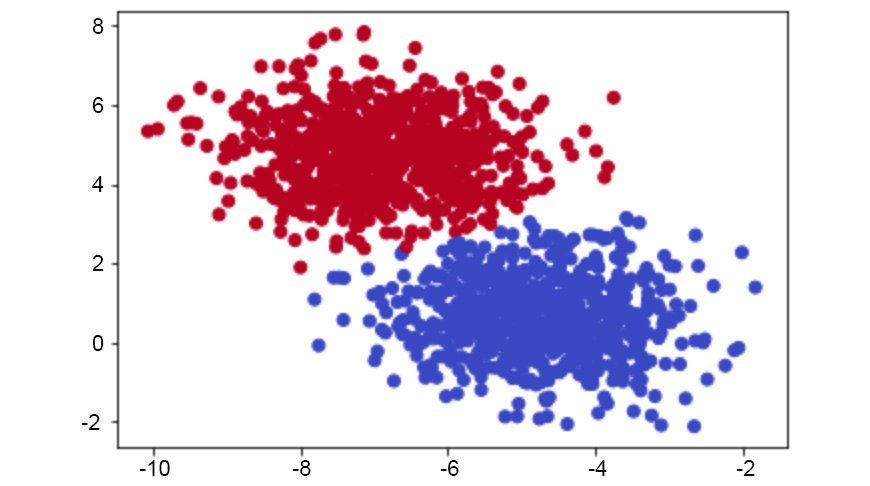Figure 1.6: Clusters in the scatterplot

2. Identify the clusters in the following scatterplot: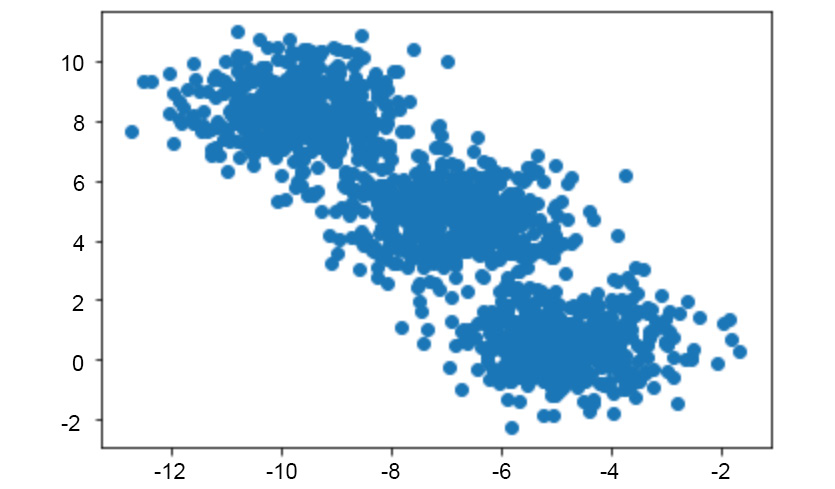Figure 1.7: Two-dimensional scatterplot

The clusters are as follows: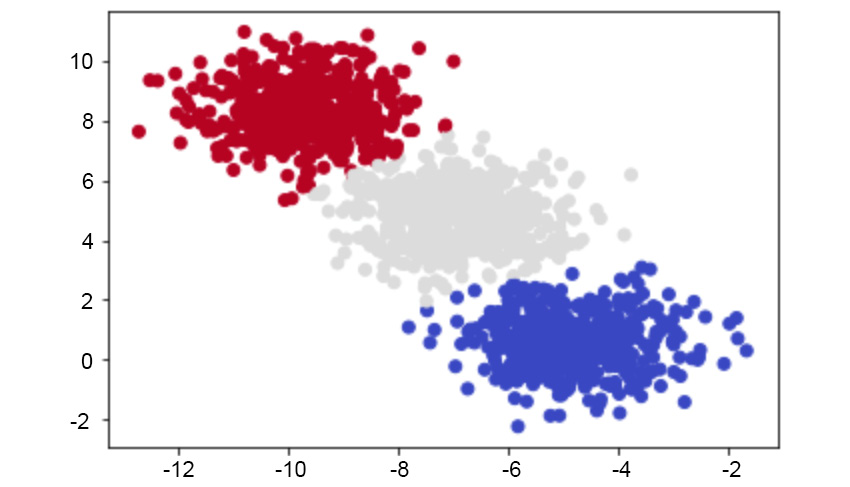Figure 1.8: Clusters in the scatterplot

3. Identify the clusters in the following scatterplot: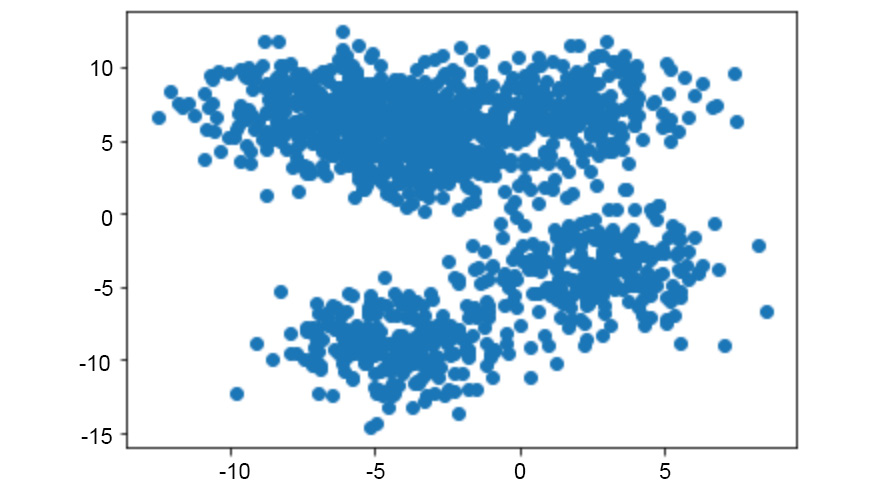Figure 1.9: Two-dimensional scatterplot

The clusters are as follows: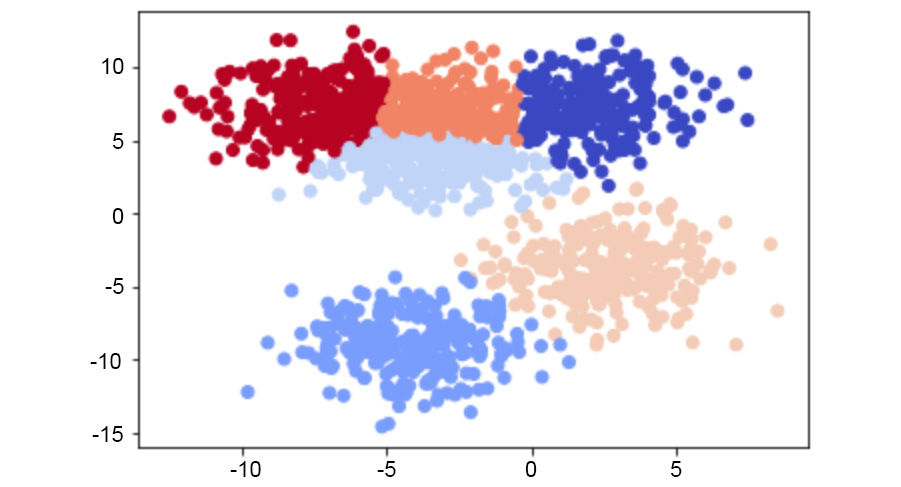Figure 1.10: Clusters in the scatterplot

Most of these examples were likely quite easy for you to understand, and that's the point. The human brain and eyes are incredible at finding patterns in the real world. Within milliseconds of viewing each plot, you could tell what fitted together and what didn't. While it is easy for you, a computer does not have the ability to see and process plots in the same manner that we do.

However, this is not always a bad thing. Look back at the preceding scatterplot. Were you able to find the six discrete clusters in the data just by looking at the plot? You probably found only three to four clusters in this scatterplot, while a computer would be able to see all six. The human brain is magnificent, but it also lacks the nuances that come with a strictly logic-based approach. Through algorithmic clustering, you will learn how to build a model that works even better than a human at these tasks.

We'll look at the clustering algorithm in the next section.

# Introduction to k-means Clustering

Hopefully, by now, you can see that finding clusters is extremely valuable in a machine learning workflow. But, how can you actually find these clusters? One of the most basic yet popular approaches is to use a cluster analysis technique called k-means clustering. The k-means clustering works by searching for k clusters in your data and the workflow is actually quite intuitive. We will start with the no-math introduction to k-means, followed by an implementation in Python. Cluster membership refers to where the points go as the algorithm processes the data. Consider it like choosing players for a sports team, where all the players are in a pool but, for each successive run, the player is assigned to a team (in this case, a cluster).

## No-Math k-means Walkthrough

The no-math algorithm for k-means clustering is pretty simple:

1. First, we'll pick "k" centroids, where "k" would be the expected distinct number of clusters. The value of k will be chosen by us and determines the type of clustering we obtain.
2. Then, we will place the "k" centroids at random places among the existing training data.
3. Next, the distance from each centroid to all the points in the training data will be calculated. We will go into detail about distance functions shortly, but for now, let's just consider it as how far points are from each other.
4. Now, all the training points will be grouped with their nearest centroid.
5. Isolating the grouped training points along with their respective centroid, calculate the mean data point in the group and move the previous centroid to the mean location.
6. This process is to be repeated until convergence or until maximum iteration limit has been achieved.

And that's it. The following image represents original raw data: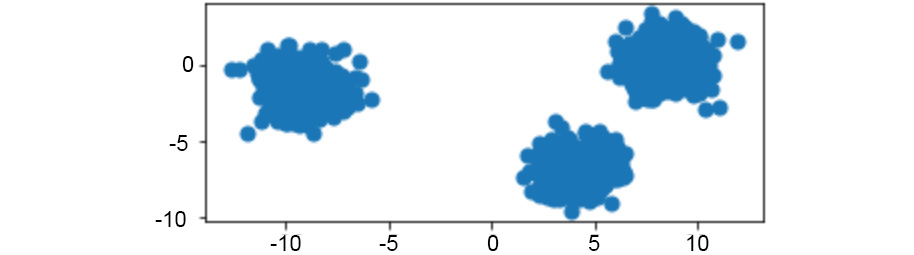Figure 1.11: Original raw data charted on x and y coordinates

Provided with the original data in the preceding image, we can visualize the iterative process of k-means by showing the predicted clusters in each step: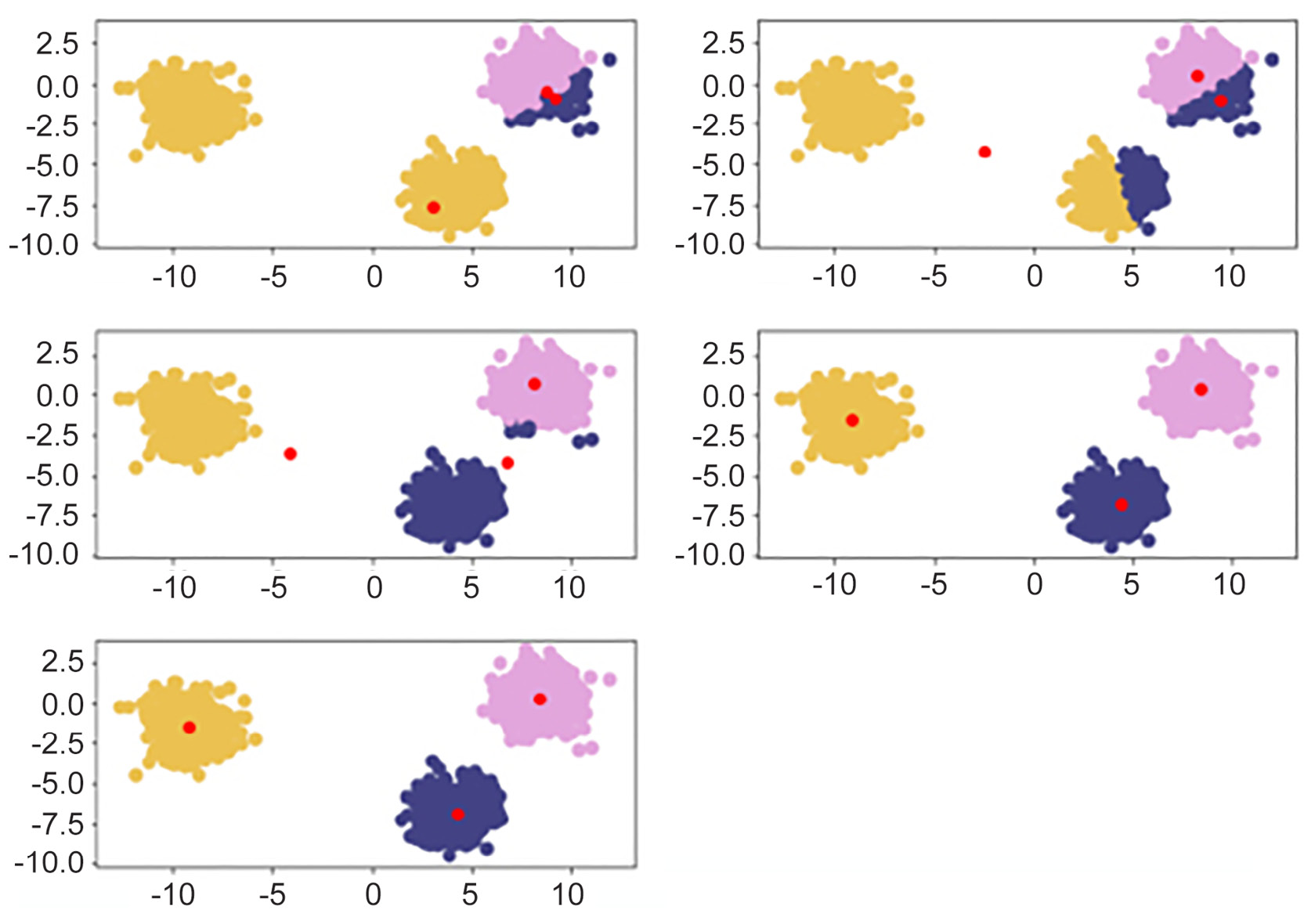Figure 1.12: Reading from left to right, red points are randomly initialized centroids, and the closest data points are assigned to groupings of each centroid

## K-means Clustering In-Depth Walkthrough

To understand k-means at a deeper level, let's walk through the example that was provided in the introduction again with some of the math that supports k-means. The most important math that underpins this algorithm is the distance function. A distance function is basically any formula that allows you to quantitatively understand how far one object is from another, with the most popular one being the Euclidean distance formula. This formula works by subtracting the respective components of each point and squaring to remove negatives, followed by adding the resulting distances and square rooting them:Figure 1.13: Euclidean distance formula

If you notice, the preceding formula holds true for data points having only two dimensions (the number of co-ordinates). A generic way of representing the preceding equation for higher-dimensional points is as follows:Figure 1.14: Euclidean distance formula for higher dimensional points

Let's see the terms involved in calculation of Euclidean distance between two points p and q in a higher dimensional space. Here, n is the number of dimensions of the two points. We compute the difference between the respective components of points p and q (pi and qi are known as the ith component of point p and q respectively) and square each of them. This squared value of the difference is summed up for all n components, and then square root of this sum is obtained. This value represents the Euclidean distance between point p and q. If you substitute n = 2 in the preceding equation, it will decompose to the equation represented in Figure 1.13.

Now coming back again to our discussion on k-means. Centroids are randomly set at the beginning as points in your n-dimensional space. Each of these centers is fed into the preceding formula as (a, b), and a point in your space is fed in as (x, y). Distances are calculated between each point and the coordinates of every centroid, with the centroid the shortest distance away chosen as the point's group.

As an example, let's pick three random centroids, an arbitrary point, and, using the Euclidean distance formula, calculate the distance from each point to the centroid:

• Random centroids: [ (2,5), (8,3), (4,5) ].
• Arbitrary point x: (0, 8).
• Distance from point to each centroid: [ 3.61, 9.43, 5.00 ].

Since the arbitrary point x is closest to the first centroid, it will be assigned to the first centroid.

## Alternative Distance Metric – Manhattan Distance

Euclidean distance is the most common distance metric for many machine learning applications and is often known colloquially as the distance metric; however, it is not the only, or even the best, distance metric for every situation. Another popular distance metric that can be used for clustering is Manhattan distance.

Manhattan distance is called as such because it mirrors the concept of traveling through a metropolis (such as New York City) that has many square blocks. Euclidean distance relies on diagonals due to its basis in Pythagorean theorem, while Manhattan distance constrains distance to only right angles. The formula for Manhattan distance is as follows:Figure 1.15: Manhattan distance formula

Here pi and qi are the ith component of points p and q, respectively. Building upon our examples of Euclidean distance, where we want to find the distance between two points, if our two points were (1,2) and (2,3), then the Manhattan distance would equal `|1-2| + |2-3| = 1 + 1 = 2`. This functionality scales to any number of dimensions. In practice, Manhattan distance may outperform Euclidean distance when it comes to high dimensional data.

## Deeper Dimensions

The preceding examples can be clearly visualized when your data is only two-dimensional. This is for convenience, to help drive the point home of how k-means works and could lead you into a false understanding of how easy clustering is. In many of your own applications, your data will likely be orders of magnitude larger to the point that it cannot be perceived by visualization (anything beyond three dimensions will be unperceivable to humans). In the previous examples, you could mentally work out a few two-dimensional lines to separate the data into its own groups. At higher dimensions, you will need to be aided by a computer to find an n-dimensional hyperplane that adequately separates the dataset. In practice, this is where clustering methods such as k-means provide significant value. The following image shows the two-dimensional, three-dimensional, and n-dimensional plots: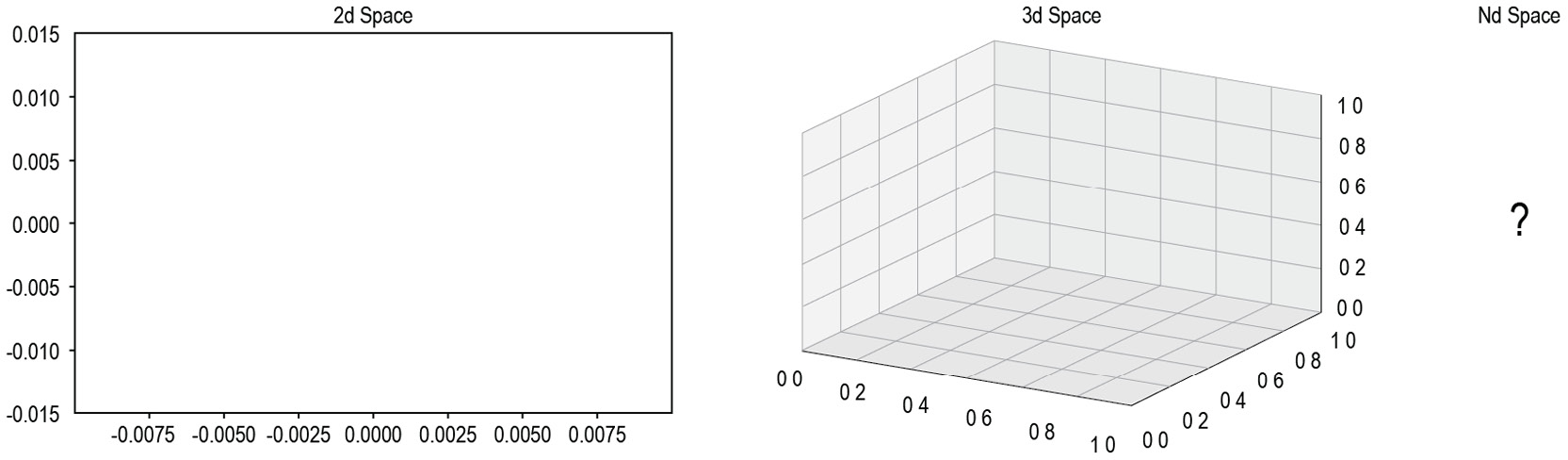Figure 1.16: Two-dimensional, three-dimensional, and n-dimensional plots

In the next exercise, we will calculate Euclidean distance. We'll build our set of tools by using the `NumPy` and `Math` Python packages. `NumPy` is a scientific computing package for Python that pre-packages common mathematical functions in highly optimized formats.

As the name implies, the `Math` package is a basic library that makes implementing foundational math building blocks, such as exponentials and square roots, much easier. By using a package such as `NumPy` or `Math`, we help cut down the time spent creating custom math functions from scratch and instead focus on developing our solutions. You will see how each of these packages is used in practice in the following exercise.

## Exercise 1.02: Calculating Euclidean Distance in Python

In this exercise, we will create an example point along with three sample centroids to help illustrate how Euclidean distance works. Understanding this distance formula is the basis for the rest of our work in clustering.

Perform the following steps to complete this exercise:

1. Open a Jupyter notebook and create a naïve formula that captures the direct math of Euclidean distance, as follows:
```import math
import numpy as np
def dist(a, b):
return math.sqrt(math.pow(a-b,2) \
+ math.pow(a-b,2))```

Note

The code snippet shown here uses a backslash ( `\` ) to split the logic across multiple lines. When the code is executed, Python will ignore the backslash, and treat the code on the next line as a direct continuation of the current line.

This approach is considered naïve because it performs element-wise calculations on your data points (slow) compared to a more real-world implementation using vectors and matrix math to achieve significant performance increases.

2. Create the data points in Python as follows:
```centroids = [ (2, 5), (8, 3), (4,5) ]
x = (0, 8)```
3. Use the formula you created to calculate the Euclidean distance in Step 1:
```# Calculating Euclidean Distance between x and centroid
centroid_distances =[]
for centroid in centroids:
print("Euclidean Distance between x {} and centroid {} is {}"\
.format(x ,centroid, dist(x,centroid)))
centroid_distances.append(dist(x,centroid))```

Note

The `#` symbol in the code snippet above denotes a code comment. Comments are added into code to help explain specific bits of logic.

The output is as follows:

```Euclidean Distance between x (0, 8) and centroid (2, 5)
is 3.605551275463989
Euclidean Distance between x (0, 8) and centroid (8, 3)
is 9.433981132056603
Euclidean Distance between x (0, 8) and centroid (4, 5) is 5.0```

The shortest distance between our point, `x`, and the centroids is `3.61`, which is equivalent to the distance between `(0, 8)` and `(2, 5)`. Since this is the minimum distance, our example point, `x`, will be assigned as a member of the first centroid's group.

In this example, our formula was used on a single point, x (0, 8). Beyond this single point, the same process will be repeated for every remaining point in your dataset until each point is assigned to a cluster. After each point is assigned, the mean point is calculated among all of the points within each cluster. The calculation of the mean among these points is the same as calculating the mean between single integers.

While there was only one point in this example, by completing this process, you have effectively assigned a point to its first cluster using Euclidean distance. We'll build upon this approach with more than one point in the following exercise.

Note

To access the source code for this specific section, please refer to https://packt.live/2VUvCuz.

You can also run this example online at https://packt.live/3ebDwpZ.

## Exercise 1.03: Forming Clusters with the Notion of Distance

It is very intuitive for our human minds to see groups of dots on a plot and determine which dots belong to discrete clusters. However, how do we ask a computer to repeat this same task? In this exercise, you'll help teach a computer an approach to forming clusters of its own with the notion of distance. We will build upon how we use these distance metrics in the next exercise:

1. Create a list of points, [ (0,8), (3,8), (3,4) ], that are assigned to cluster one:
`cluster_1_points =[ (0,8), (3,8), (3,4) ]`
2. To find the new centroid among your list of points, calculate the mean point between all of the points. Calculation of the mean scales to infinite points, as you simply add the integers at each position and divide by the total number of points. For example, if your two points are (0,1,2) and (3,4,5), the mean calculation would be [ (0+3)/2, (1+4)/2, (2+5)/2 ]:
```mean =[ (0+3+3)/3, (8+8+4)/3 ]
print(mean)```

The output is as follows:

`[2.0, 6.666666666666667]`

After a new centroid is calculated, repeat the cluster membership calculation we looked at in Exercise 1.02, Calculating Euclidean Distance in Python, and then repeat the previous two steps to find the new cluster centroid. Eventually, the new cluster centroid will be the same as the centroid before the cluster membership calculation and the exercise will be complete. How many times this repeats depends on the data you are clustering.

Once you have moved the centroid location to the new mean point of (2, 6.67), you can compare it to the initial list of centroids you entered the problem with. If the new mean point is different than the centroid that is currently in your list, you will have to go through another iteration of the preceding two exercises. Once the new mean point you calculate is the same as the centroid you started the problem with, you have completed a run of k-means and reached a point called convergence. However, in practice, sometimes the number of iterations required to reach convergence is very large and such large computations may not be practically feasible. In such cases, we need to set a maximum limit to the number of iterations. Once this iteration limit is reached, we stop further processing.

Note

To access the source code for this specific section, please refer to https://packt.live/3iJ3JiT.

You can also run this example online at https://packt.live/38CCpOG.

In the next exercise, we will implement k-means from scratch. To do this, we will start employing common packages from the Python ecosystem that will serve as building blocks for the rest of your career. One of the most popular machine learning libraries is called scikit-learn (https://scikit-learn.org/stable/user_guide.html), which has many built-in algorithms and functions to support your understanding of how the algorithms work. We will also be using functions from SciPy (https://docs.scipy.org/doc/scipy/reference/), which is a package much like NumPy and abstracts away basic scientific math functions that allow for more efficient deployment. Finally, the next exercise will introduce `matplotlib` (https://matplotlib.org/3.1.1/contents.html), which is a plotting library that creates graphical representations of the data you are working with.

## Exercise 1.04: K-means from Scratch – Part 1: Data Generation

The next two exercises focus on the creation of exercise data and the implementation of k-means from scratch on your training data. This exercise relies on scikit-learn, an open source Python package that enables the fast prototyping of popular machine learning models. Within scikit-learn, we will be using the `datasets` functionality to create a synthetic blob dataset. In addition to harnessing the power of scikit-learn, we will also rely on Matplotlib, a popular plotting library for Python that makes it easy for us to visualize our data. To do this, perform the following steps:

1. Import the necessary libraries:
```from sklearn.datasets import make_blobs
from sklearn.cluster import KMeans
import matplotlib.pyplot as plt
import numpy as np
import math
np.random.seed(0)
%matplotlib inline```

Note

You can find more details on the `KMeans` library at https://scikit-learn.org/stable/modules/clustering.html#k-means.

2. Generate a random cluster dataset to experiment on X = coordinate points, y = cluster labels, and define random centroids. We will achieve this with the `make_blobs` function that we imported from `sklearn.datasets`, which, as the name implies, generates blobs of data points.
```X, y = make_blobs(n_samples=1500, centers=3, \
n_features=2, random_state=800)
centroids = [[-6,2],[3,-4],[-5,10]]```

Here the `n_samples` parameter determines the total number of data points generated by the blobs. The `centers` parameter determines the number of centroids for the blob. The `n_feature` attribute defines the number of dimensions generated by the dataset. Here, the data will be two dimensional.

In order to generate the same data points in all the iterations (which in turn are generated randomly) for reproducibility of results, we set the `random_state` parameter to `800`. Different values of the `random_state` parameter would yield different results. If we do not set the `random_state` parameter, each time on execution we will obtain different results.

3. Print the data:
`X`

The output is as follows:

```array([[-3.83458347,  6.09210705],
[-4.62571831,  5.54296865],
[-2.87807159, -7.48754592],
...,
[-3.709726  , -7.77993633],
[-8.44553266, -1.83519866],
[-4.68308431,  6.91780744]])```
4. Plot the coordinate points using the scatterplot functionality we imported from `matplotlib.pyplot`. This function takes input lists of points and presents them graphically for ease of understanding. Please review the `matplotlib` documentation if you want to explore the parameters provided at a deeper level:
```plt.scatter(X[:, 0], X[:, 1], s=50, cmap='tab20b')
plt.show()```

The plot appears as follows: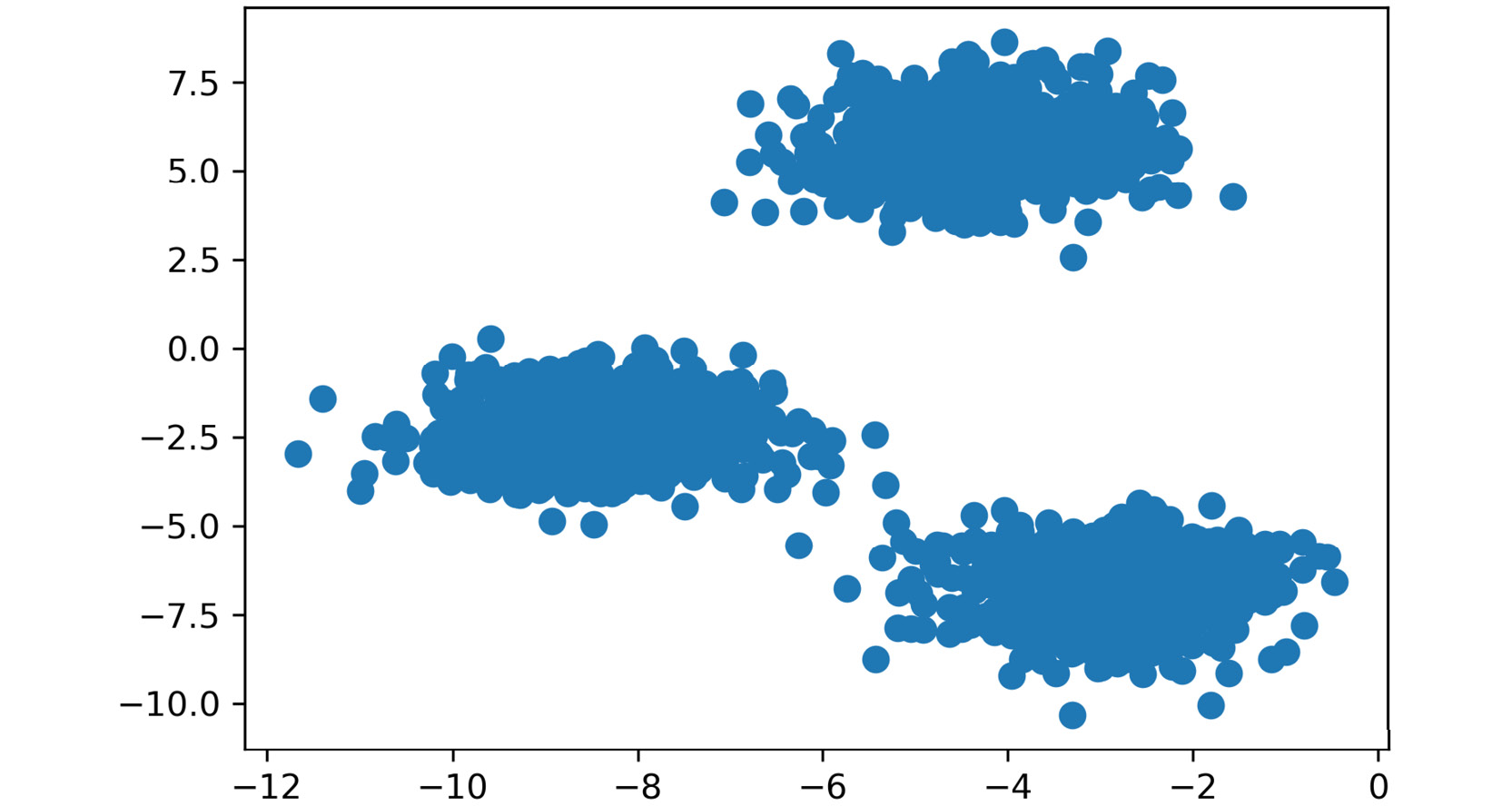Figure 1.17: Plot of the coordinates

5. Print the array of `y`, which is the labels provided by scikit-learn and serves as the ground truth for comparison.

Note

These labels will not be known to us in practice. This is just for us to cross verify our clustering in later stages.

Use the following code to print the array:

`y`

The output is as follows:

`array([2, 2, 1, ..., 1, 0, 2])`
6. Plot the coordinate points with the correct cluster labels:
```plt.scatter(X[:, 0], X[:, 1], c=y,s=50, cmap='tab20b')
plt.show()```

The plot appears as follows: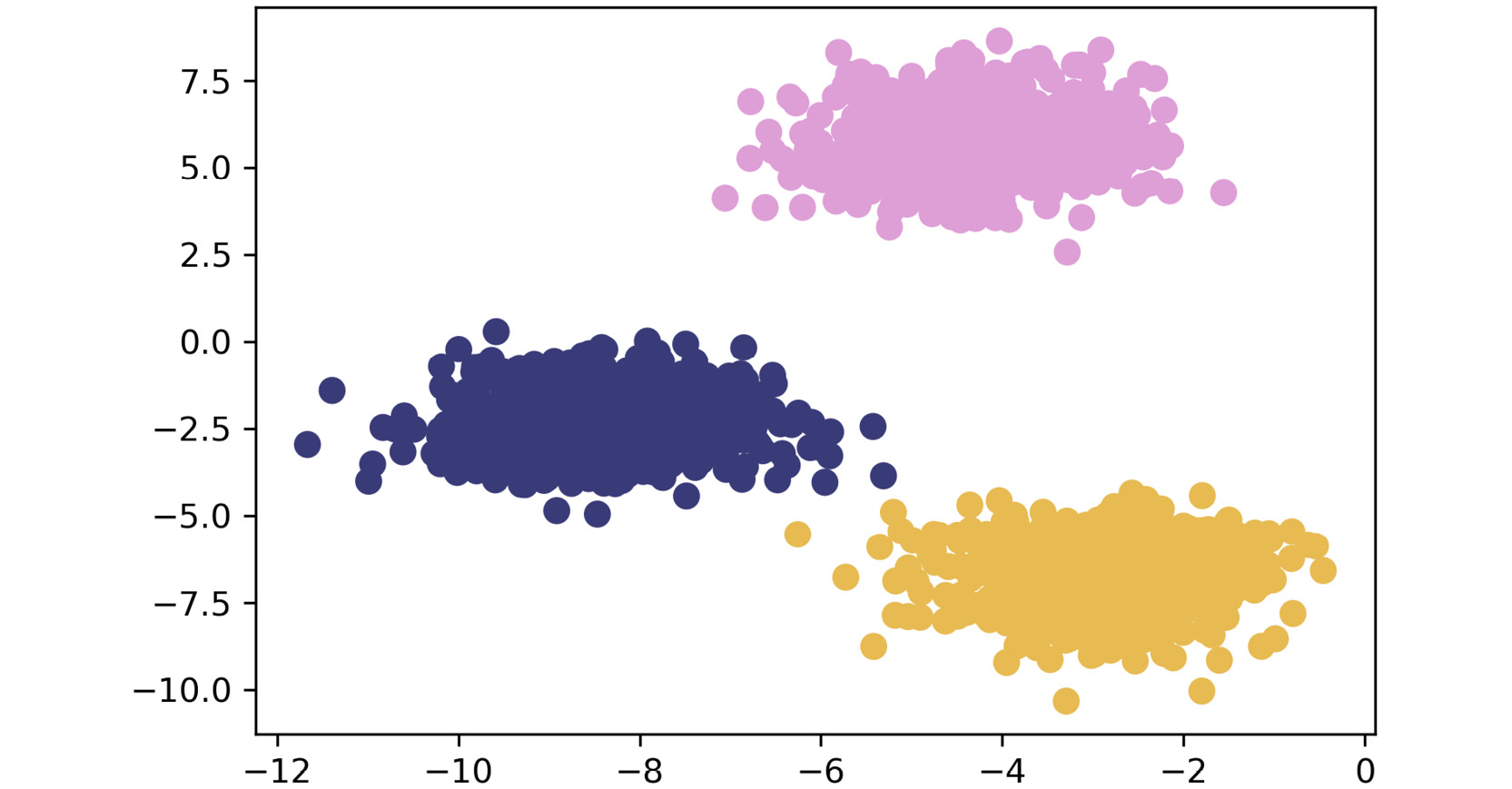Figure 1.18: Plot of the coordinates with correct cluster labels

By completing the preceding steps, you have generated the data and visually explored how it is put together. By visualizing the ground truth, you have established a baseline that provides a relative metric for algorithm accuracy.

Note

To access the source code for this specific section, please refer to https://packt.live/2JM8Q1S.

You can also run this example online at https://packt.live/3ecjKdT.

With data in hand, in the next exercise, we'll continue by building your unsupervised learning toolset with an optimized version of the Euclidean distance function from the `SciPy` package, `cdist`. You will compare a non-vectorized, clearly understandable version of the approach with `cdist`, which has been specially tweaked for maximum performance.

## Exercise 1.05: K-means from Scratch – Part 2: Implementing k-means

Let's recreate these results on our own. We will go over an example implementing this with some optimizations.

Note

This exercise is a continuation of the previous exercise and should be performed in the same Jupyter notebook.

For this exercise, we will rely on SciPy, a Python package that allows easy access to highly optimized versions of scientific calculations. In particular, we will be implementing Euclidean distance with `cdist`, the functionally of which replicates the barebones implementation of our distance metric in a much more efficient manner. Follow these steps to complete this exercise:

1. The basis of this exercise will be comparing a basic implementation of Euclidean distance with an optimized version provided in SciPy. First, import the optimized Euclidean distance reference:
`from scipy.spatial.distance import cdist`
2. Identify a subset of `X` you want to explore. For this example, we are only selecting five points to make the lesson clearer; however, this approach scales to any number of points. We chose points 105-109, inclusive:
`X[105:110]`

The output is as follows:

```array([[-3.09897933,  4.79407445],
[-3.37295914, -7.36901393],
[-3.372895  ,  5.10433846],
[-5.90267987, -3.28352194],
[-3.52067739,  7.7841276 ]])```
3. Calculate the distances and choose the index of the shortest distance as a cluster:
```"""
Finds distances from each of 5 sampled points to all of the centroids
"""
for x in X[105:110]:
calcs = cdist(x.reshape([1,-1]),centroids).squeeze()
print(calcs, "Cluster Membership: ", np.argmin(calcs))```

Note

The triple-quotes ( `"""` ) shown in the code snippet above are used to denote the start and end points of a multi-line code comment. Comments are added into code to help explain specific bits of logic.

The preceding code will result in the following output:

```[4.027750355981394, 10.70202290628413, 5.542160268055164]
Cluster Membership:  0
[9.73035280174993, 7.208665829113462, 17.44505393393603]
Cluster Membership:  1
[4.066767506545852, 11.113179986633003, 5.1589701124301515]
Cluster Membership:  0
[5.284418164665783, 8.931464028407861, 13.314157359115697]
Cluster Membership:  0
[6.293105164930943, 13.467921029846712, 2.664298385076878]
Cluster Membership:  2```
4. Define the `k_means` function as follows and initialize the k-centroids randomly. Repeat this process until the difference between the new/old `centroids` equals `0`, using the `while` loop:

Exercise1.04-Exercise1.05.ipynb

```def k_means(X, K):
# Keep track of history so you can see K-Means in action
centroids_history = []
labels_history = []
rand_index = np.random.choice(X.shape, K)
centroids = X[rand_index]
centroids_history.append(centroids)```

Note

Do not break this code, as it might lead to an error.

5. Zip together the historical steps of centers and their labels:
```history = zip(centers_hist, labels_hist)
for x, y in history:
plt.figure(figsize=(4,3))
plt.scatter(X[:, 0], X[:, 1], c=y, s=50, cmap='tab20b');
plt.scatter(x[:, 0], x[:, 1], c='red')
plt.show()```

The following plots may differ from what you can see if we haven't set the random seed. The first plot looks as follows: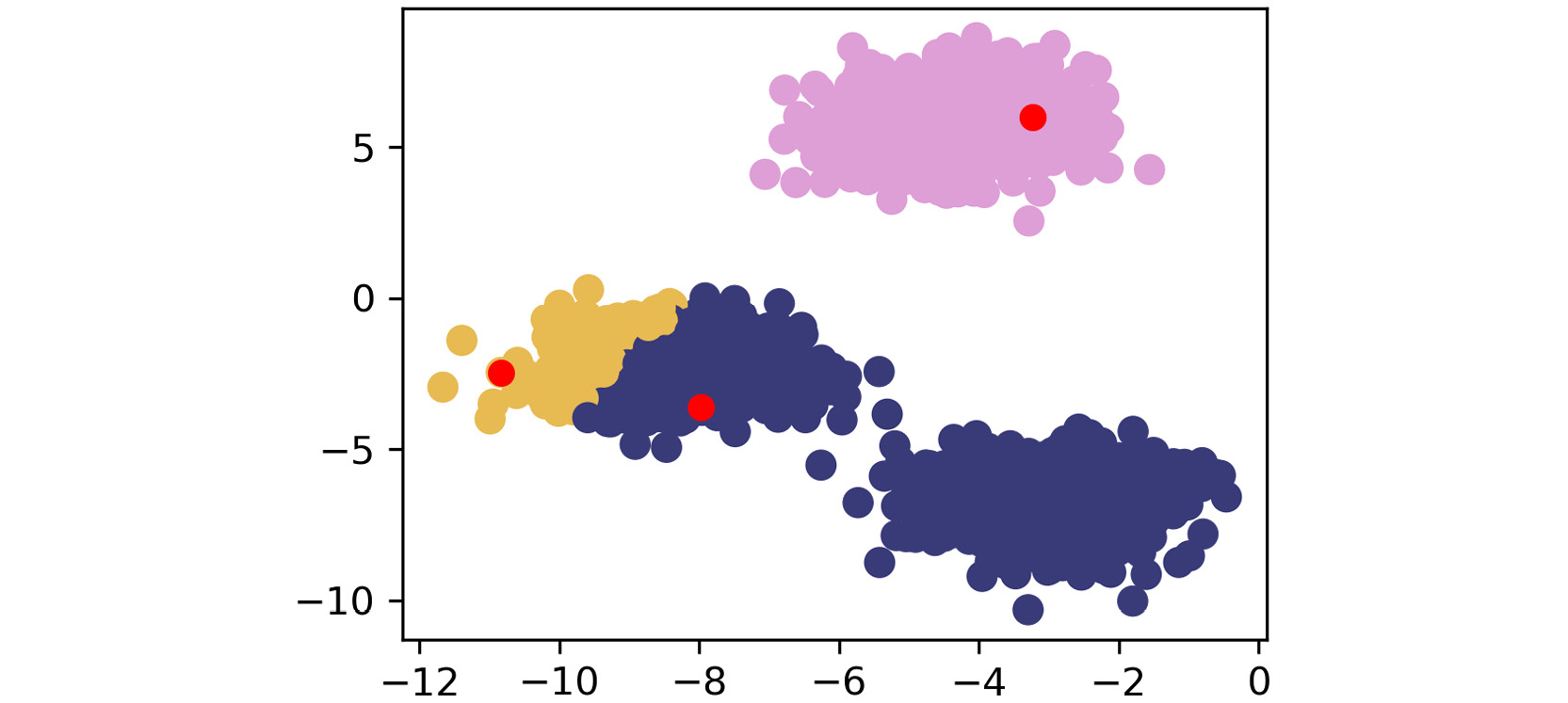Figure 1.19: First scatterplot

The second plot appears as follows: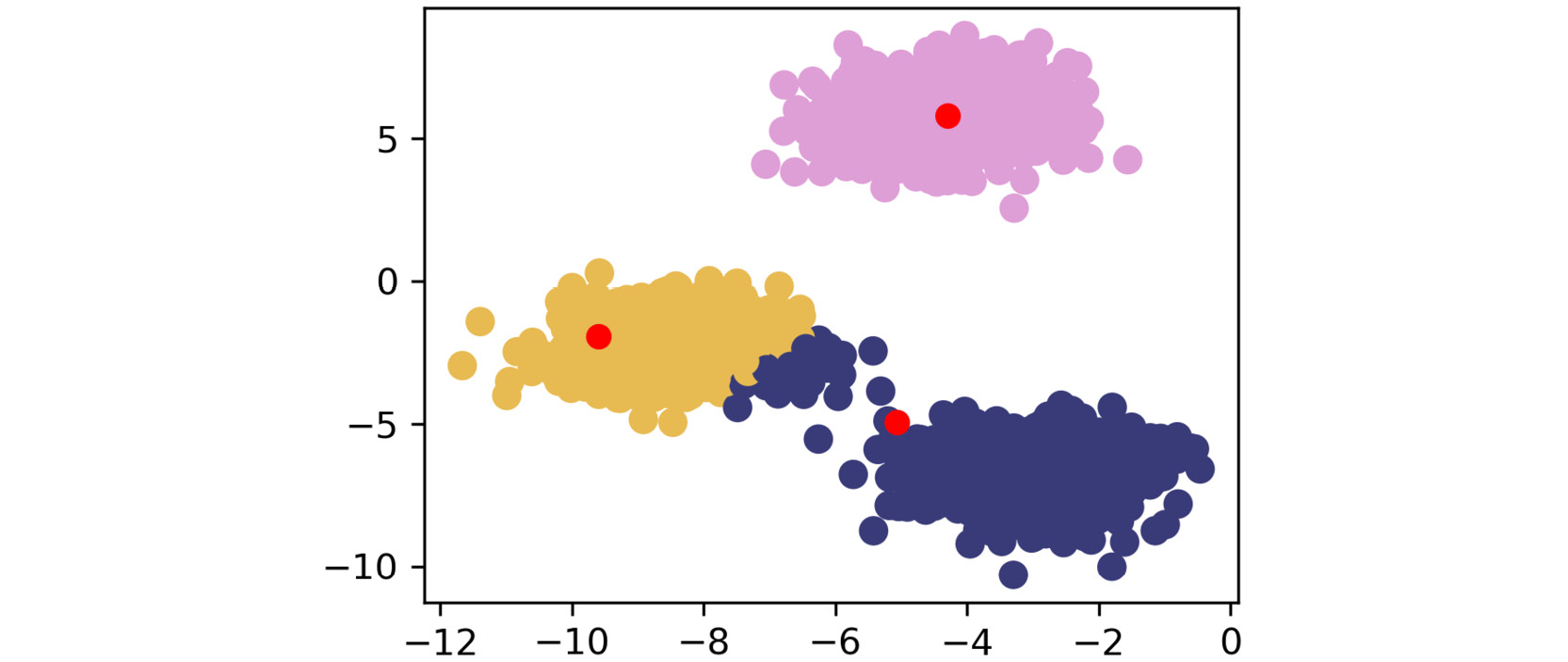Figure 1.20: Second scatterplot

The third plot appears as follows: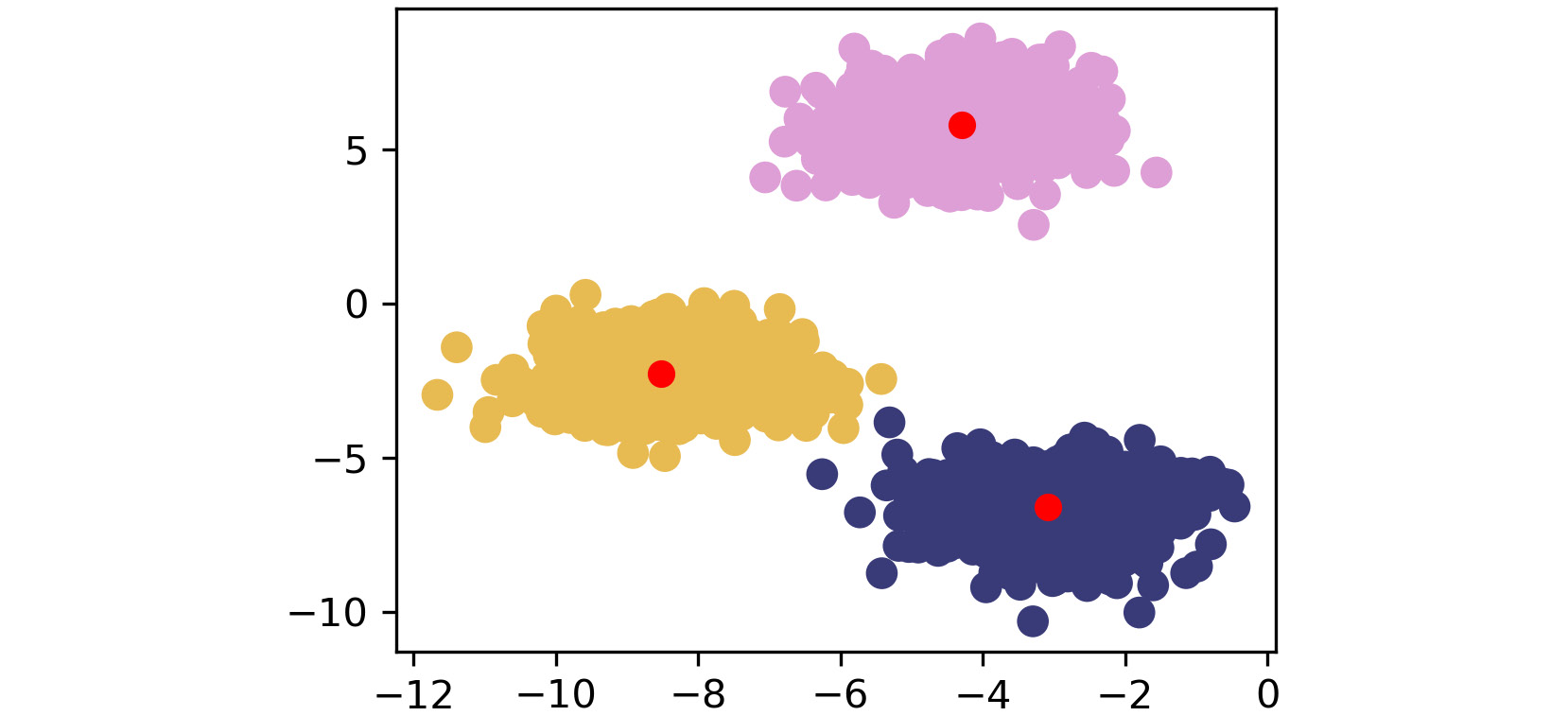Figure 1.21: Third scatterplot

The fourth plot appears as follows: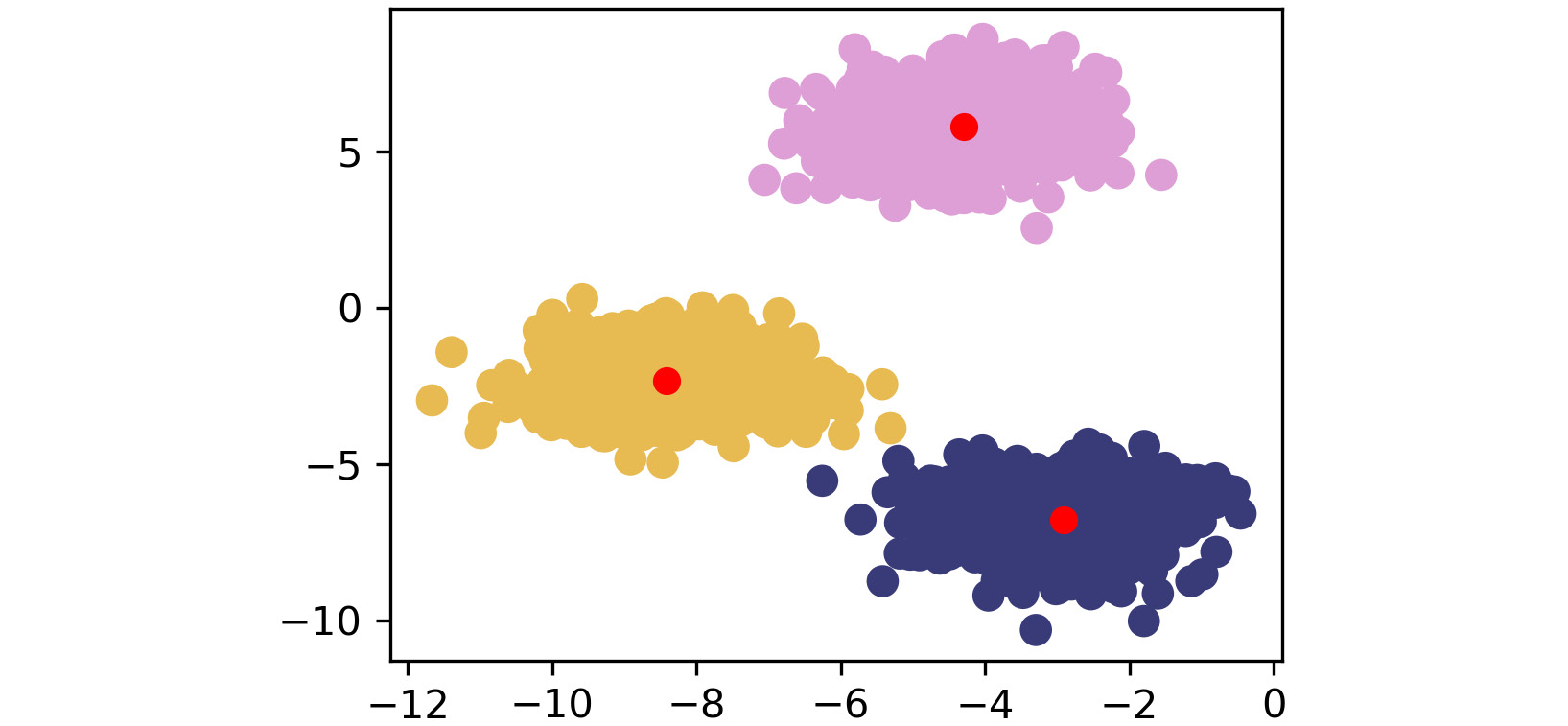Figure 1.22: Fourth scatterplot

The fifth plot looks as follows: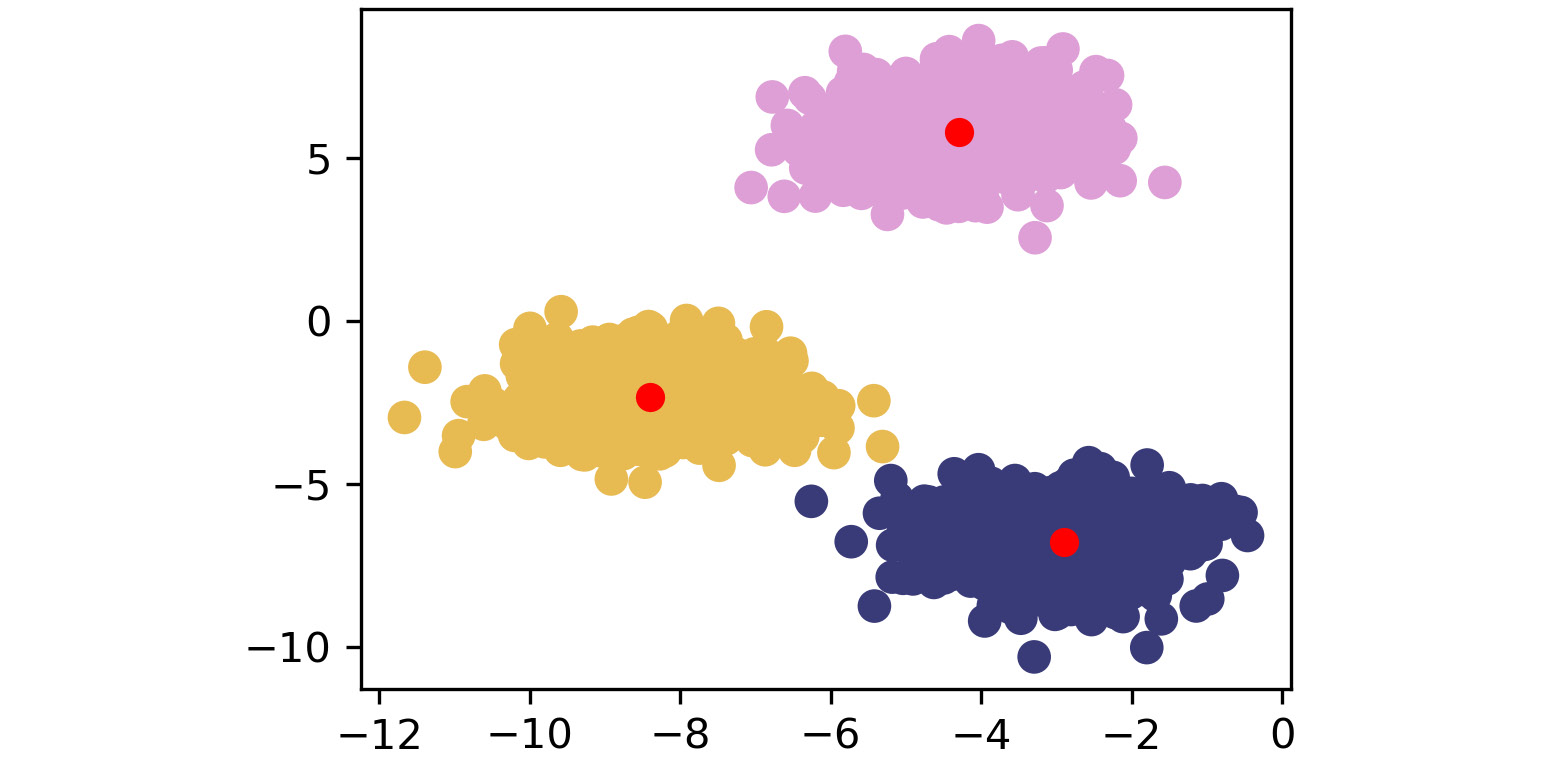Figure 1.23: Fifth scatterplot

As shown by the preceding images, k-means takes an iterative approach to refine optimal clusters based on distance. The algorithm starts with random initialization of centroids and, depending on the complexity of the data, quickly finds the separations that make the most sense.

Note

To access the source code for this specific section, please refer to https://packt.live/2JM8Q1S.

You can also run this example online at https://packt.live/3ecjKdT.

## Clustering Performance – Silhouette Score

Understanding the performance of unsupervised learning methods is inherently much more difficult than supervised learning methods because there is no ground truth available. For supervised learning, there are many robust performance metrics—the most straightforward of these being accuracy in the form of comparing model-predicted labels to actual labels and seeing how many the model got correct. Unfortunately, for clustering, we do not have labels to rely on and need to build an understanding of how "different" our clusters are. We achieve this with the silhouette score metric. We can also use silhouette scores to find the optimal "K" numbers of clusters for our unsupervised learning methods.

The silhouette metric works by analyzing how well a point fits within its cluster. The metric ranges from -1 to 1. If the average silhouette score across your clustering is one, then you will have achieved perfect clusters and there will be minimal confusion about which point belongs where. For the plots in the previous exercise, the silhouette score will be much closer to one since the blobs are tightly condensed and there is a fair amount of distance between each blob. This is very rare, though; the silhouette score should be treated as an attempt at doing the best you can, since hitting one is highly unlikely. If the silhouette score is positive, it means that a point is closer to the assigned cluster than it is to the neighboring clusters. If the silhouette score is 0, then a point lies on the boundary between the assigned cluster and the next closest cluster. If the silhouette score is negative, then it indicates that a given point is assigned to an incorrect cluster, and the given point in fact likely belongs to a neighboring cluster.

Mathematically, the silhouette score calculation is quite straightforward and is obtained using the Simplified Silhouette Index (SSI):

`SSIi = bi - ai/ max(ai, bi)`

Here ai is the distance from point i to its own cluster centroid, and bi is the distance from point i to the nearest cluster centroid.

The intuition captured here is that ai represents how cohesive the cluster of point i' is as a clear cluster, and bi represents how far apart the clusters lie. We will use the optimized implementation of `silhouette_score` in scikit-learn in Activity 1.01, Implementing k-means Clustering. Using it is simple and only requires that you pass in the feature array and the predicted cluster labels from your k-means clustering method.

In the next exercise, we will use the `pandas` library (https://pandas.pydata.org/pandas-docs/stable/) to read a CSV file. Pandas is a Python library that makes data wrangling easier through the use of DataFrames. If you look back at the arrays you built with NumPy, you probably noticed that the resulting data structures are quite unwieldly. To extract subsets from the data, you had to index using brackets and specific numbers of rows. Instead of this approach, pandas allows an easier-to-understand approach to moving data around and getting it into the format necessary for unsupervised learning and other machine learning techniques.

Note

To read data in Python, you will use `variable_name = pd.read_csv('file_name.csv', header=None)`

Here, the parameter `header = None` explicitly mentions that there is no presence of column names. If your file contains column names, then retain those default values. Also, if you specify `header = None` for a file which contains column names, Pandas will treat the row containing names of column as the row containing data only.

## Exercise 1.06: Calculating the Silhouette Score

In this exercise, we will calculate the silhouette score of a dataset with a fixed number of clusters. For this, we will use the seeds dataset, which is available at https://packt.live/2UQA79z. The following note outlines more information regarding this dataset, in addition to further exploration in the next activity. For the purpose of this exercise, please disregard the specific details of what this dataset is comprised of as it is of greater importance to learn about the silhouette score. As we go into the next activity, you will gain more context as needed to create a smart machine learning system. Follow these steps to complete this exercise:

Note

This dataset is sourced from https://archive.ics.uci.edu/ml/datasets/seeds. It can be accessed at https://packt.live/2UQA79z

Citation: Contributors gratefully acknowledge support of their work by the Institute of Agrophysics of the Polish Academy of Sciences in Lublin.

1. Load the seeds data file using pandas, a package that makes data wrangling much easier through the use of DataFrames:
```import pandas as pd
import numpy as np
import matplotlib.pyplot as plt
from sklearn.metrics import silhouette_score
from scipy.spatial.distance import cdist
np.random.seed(0)
2. Separate the `X` features, since we want to treat this as an unsupervised learning problem:
`X = seeds[['A','P','C','LK','WK','A_Coef','LKG']]`
3. Bring back the `k_means` function we made earlier for reference:

Exercise 1.06.ipynb

```def k_means(X, K):
# Keep track of history so you can see K-Means in action
centroids_history = []
labels_history = []
rand_index = np.random.choice(X.shape, K)
centroids = X[rand_index]
centroids_history.append(centroids)```
4. Convert our seeds `X` feature DataFrame into a `NumPy` matrix:
`X_mat = X.values`
5. Run our `k_means` function on the seeds matrix:
```centroids, labels, centroids_history, labels_history = \
k_means(X_mat, 3)```
6. Calculate the silhouette score for the `Area ('A')` and `Length of Kernel ('LK')` columns:
`silhouette_score(X[['A','LK']], labels)`

The output should be similar to the following:

`0.5875704550892767`

In this exercise, we calculated the silhouette score for the `Area ('A')` and `Length of Kernel ('LK')` columns of the seeds dataset. We will use this technique in the next activity to determine the performance of our k-means clustering algorithm.

Note

To access the source code for this specific section, please refer to https://packt.live/2UOqW9H.

You can also run this example online at https://packt.live/3fbtJ4y.

## Activity 1.01: Implementing k-means Clustering

You are implementing a k-means clustering algorithm from scratch to prove that you understand how it works. You will be using the seeds dataset provided by the UCI ML repository. The seeds dataset is a classic in the data science world and contains features of wheat kernels that are used to predict three different types of wheat species. The download location can be found later in this activity.

For this activity, you should use Matplotlib, NumPy, scikit-learn metrics, and pandas.

The following seeds data features are provided for reference:

```1. area (A),
2. perimeter (P)
3. compactness (C)
4. length of kernel (LK)
5. width of kernel (WK)
6. asymmetry coefficient (A_Coef)
7. length of kernel groove (LKG)```

The aim here is to truly understand how k-means works. To do so, you need to take what you have learned in the previous sections and implement k-means from scratch in Python.

1. Using `NumPy` or the `math` package and the Euclidean distance formula, write a function that calculates the distance between two coordinates.
2. Write a function that calculates the distance from the centroids to each of the points in your dataset and returns the cluster membership.
3. Write a k-means function that takes in a dataset and the number of clusters (K) and returns the final cluster centroids, as well as the data points that make up that cluster's membership. After implementing k-means from scratch, apply your custom algorithm to the seeds dataset, which is located here: https://packt.live/2Xh2FdS.

Note

This dataset is sourced from https://archive.ics.uci.edu/ml/datasets/seeds. It can be accessed at https://packt.live/2Xh2FdS.

UCI Machine Learning Repository [http://archive.ics.uci.edu/ml]. Irvine, CA: University of California, School of Information and Computer Science.

Citation: Contributors gratefully acknowledge support of their work by the Institute of Agrophysics of the Polish Academy of Sciences in Lublin.

4. Remove the classes supplied in this dataset and see whether your k-means algorithm can group the different wheat species into their proper groups just based on plant characteristics!
5. Calculate the silhouette score using the scikit-learn implementation.

In completing this exercise, you have gained hands-on experience of tuning a k-means clustering algorithm for a real-world dataset. The seeds dataset is seen as a classic "hello world"-type problem in the data science space and is helpful for testing foundational techniques. Your final clustering algorithm should do a decent job of finding the three clusters of wheat species types that exist in the data, as follows: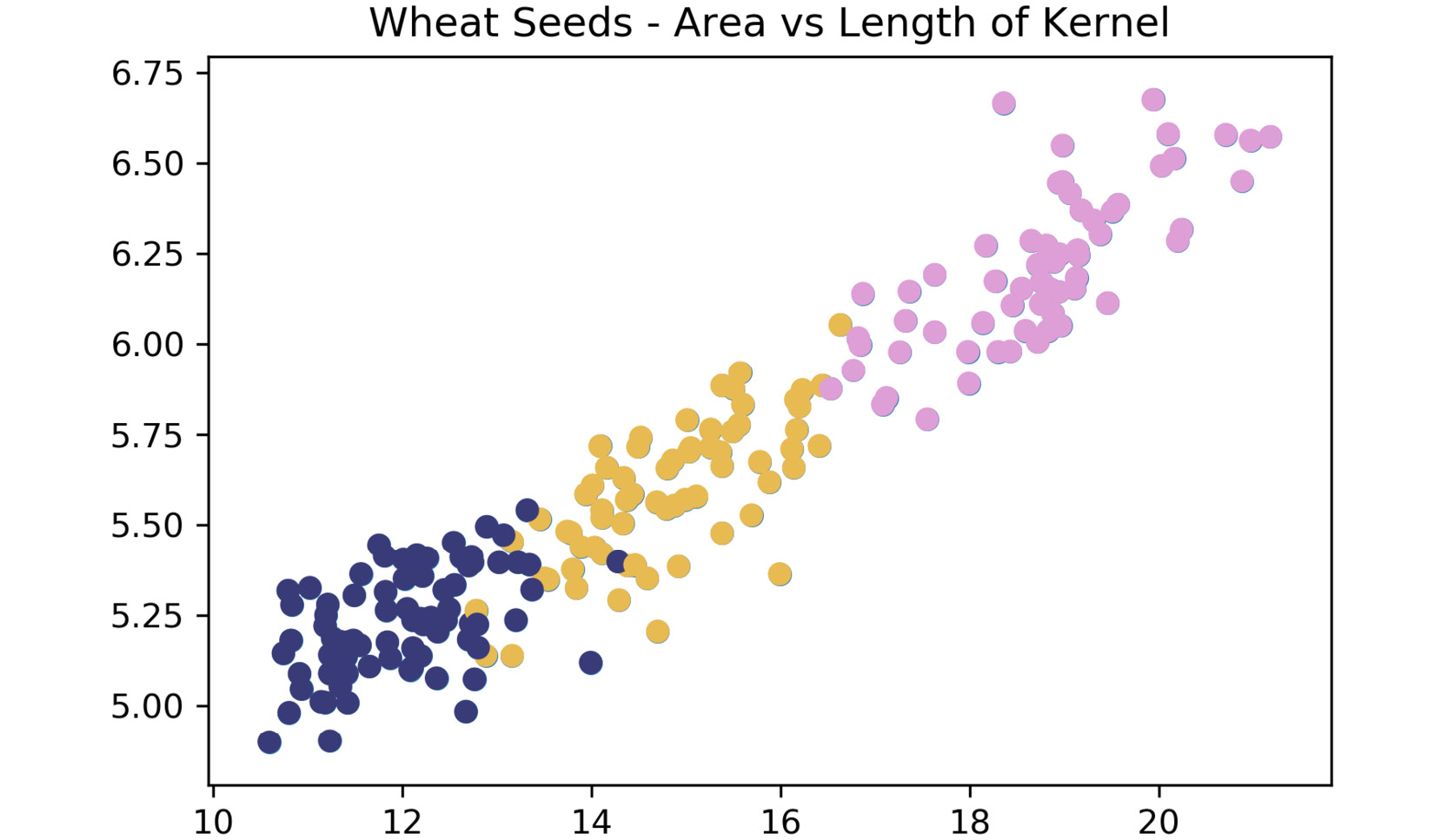Figure 1.24: Expected plot of three clusters of wheat species

Note

The solution for this activity can be found via this link.

# Summary

In this chapter, we have explored what clustering is and why it is important in a variety of data challenges. Building upon this foundation of clustering knowledge, you implemented k-means, which is one of the simplest, yet most popular, methods of unsupervised learning. If you have reached this summary and can repeat what k-means does step by step to a friend, then you're ready to move on to more complex forms of clustering.

From here, we will be moving on to hierarchical clustering, which, in one configuration, reuses the centroid learning approach that we used in k-means. We will build upon this approach by outlining additional clustering methodologies and approaches in the next chapter.

• Aaron Jones

Aaron Jones is a full-time senior data scientist and consultant. He has built models and data products while working in retail, media, and environmental science. Aaron is based in Seattle, Washington and has a particular interest in clustering algorithms, natural language processing, and Bayesian statistics.

Browse publications by this author
• Christopher Kruger

Christopher Kruger is a practicing data scientist and AI researcher. He has managed applied machine learning projects across multiple industries while mentoring junior team members on best practices. His primary focus is on pushing both business practicality as well as academic rigor in every project. Chris is currently developing research in the computer vision space.

Browse publications by this author
• Benjamin Johnston

Benjamin Johnston is a senior data scientist for one of the world's leading data-driven MedTech companies and is involved in the development of innovative digital solutions throughout the entire product development pathway, from problem definition to solution research and development, through to final deployment. He is currently completing his Ph.D. in machine learning, specializing in image processing and deep convolutional neural networks. He has more than 10 years of experience in medical device design and development, working in a variety of technical roles, and holds first-class honors bachelor's degrees in both engineering and medical science from the University of Sydney, Australia.

Browse publications by this author